# PSEB 11th Class Physics Solutions Chapter 14 Oscillations

Punjab State Board PSEB 11th Class Physics Book Solutions Chapter 14 Oscillations Textbook Exercise Questions and Answers.

## PSEB Solutions for Class 11 Physics Chapter 14 Oscillations

PSEB 11th Class Physics Guide Oscillations Textbook Questions and Answers

Question 1.
Which of the following examples represent periodic motion?
(a) A swimmer completing one (return) trip from one bank of a river to the other and back.
(b) A freely suspended bar magnet displaced from its N-S direction and released.
(c) A hydrogen molecule rotating about its center of mass.
(d) An arrow released from a bow.
Solution:
(b) and (c)
Explanations :
(a) The swimmer’s motion is not periodic. The motion of the swimmer between the banks of a river is back and forth. However, it does not have a definite period. This is because the time taken by the swimmer during his back and forth journey may not be the same.

(b) The motion of a freely-suspended magnet, if displaced from its N-S direction and released, is periodic. This is because the magnet oscillates about its position with a definite period of time.

(c) When a hydrogen molecule rotates about its center of mass, it comes to the same position again and again after an equal interval of time. Such motion is periodic.

(d) An arrow released from a bow moves only in the forward direction. It does not come backward. Hence, this motion is not a periodic.

Question 2.
Which of the following examples represent (nearly) simple harmonic motion and which represent periodic but not simple harmonic motion?
(a) the rotation of earth about its axis.
(b) motion of an oscillating mercury column in a 17-tube.
(c) motion of a ball bearing inside a smooth curved bowl, when released from a point slightly above the lowermost point.
(d) general vibrations of a polyatomic molecule about its equilibrium position.
Solution:
(b) and (c) are SHMs; (a) and (d) are periodic, but not SHMs
Explanations :
(a) During its rotation about its axis, earth comes to the same position again and again in equal intervals of time. Hence, it is a periodic motion. However, this motion is not simple harmonic. This is because earth does not have a to and fro motion about its axis.

(b) An oscillating mercury column in a [/-tube is simple harmonic. This is because the mercury moves to and fro on the same path, about the fixed position, with a certain period of time.

(c) The ball moves to and fro about the lowermost point of the bowl when released. Also, the ball comes back to its initial position in the same period of time, again and again. Hence, its motion is periodic as well as simple harmonic.

(d) A polyatomic molecule has many natural frequencies of oscillation. Its vibration is the superposition of individual simple harmonic motions of a number of different molecules. Hence, it is not simple harmonic, but periodic.Question 3.
Figure depicts four x-t plots for linear motion of a particle. Which of the plots represents periodic motion? What is the period of motion (in case of periodic motion)?
(a)(b)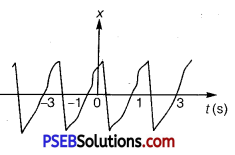(c)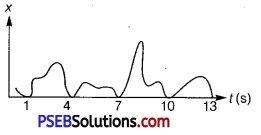(d)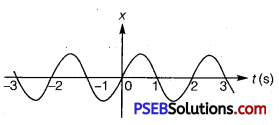Solution:
(b) and (d) are periodic
Explanation :
(a) It is not a periodic motion. This represents a unidirectional, linear uniform motion. There is no repetition of motion in this case.
(b) In this case, the motion of the particle repeats itself after 2 s. Hence, it is a periodic motion, having a period of 2 s.
(c) It is not a periodic motion. This is because the particle repeats the motion in one position only. For a periodic motion, the entire motion of the particle must be repeated in equal intervals of time. In this case, the motion of the particle repeats itself after 2 s. Hence, it is a periodic motion, having a period of 2 s.

Question 4.
Which of the following functions of time represent (a) simple ‘ harmonic, (b) periodic but not simple harmonic, and (c) non¬periodic motion? Give period for each case of periodic motion (a is any positive constant):
(a) sin ωt – cos ωt
(b) sin 3ωt
(c) 3cos($$\pi / 4$$ -2ωt)
(d) cos ωt +cos 3 ωt+cos 5ωt
(e) exp(-ω2t2)
(f) 1+ ωt+ω2t
Solution:
(a) SHM
The given function is: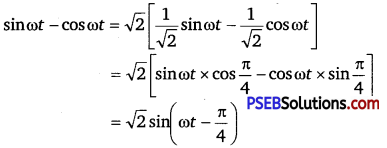This function represents SHM as it can be written in the form: a sin (ωt +Φ)
Its period is: $$\frac{2 \pi}{\omega}$$

(b) Periodic, but not SHM The given function is:
sin3 ωt = $$\frac{1}{4}$$ [3sinωt -sin3ωt] (∵ sin3θ = 3sinθ – 4sin3 θ)
The terms sin cot and sin 3 ωt individually represent simple harmonic motion (SHM). However, the superposition of two SHM is periodic and not simple harmonic.

Period of$$\frac{3}{4}$$sin ωt = $$\frac{2 \pi}{\omega}$$ = T
Period of$$\frac{1}{4}$$sin3ωt = $$\frac{2 \pi}{3 \omega}$$ = T’ = $$\frac{T}{3}$$
Thus, period of the combination
= Minimum time after which the combined function repeats
= LCM of T and $$\frac{T}{3}$$ = T
Its period is 2 $$\pi / \omega$$

(c) SHM
The given function is:
3 cos $$\left[\frac{\pi}{4}-2 \omega t\right]$$ = 3 cos $$\left[2 \omega t-\frac{\pi}{4}\right]$$
This function represents simple harmonic motion because it can be written in the form:
acos(ωt +Φ)

Its period is :
$$\frac{2 \pi}{2 \omega}=\frac{\pi}{\omega}$$

(d) Periodic, but not SHM
The given function is cosωt +cos3ωt +cos5ωt. Each individual cosme function represents SHM. However, the superposition of threc simple harmonic motions is periodic, but not simple harmonic.

cosωt represents SHM with period = $$\frac{2 \pi}{\omega}$$ T (say)
cos 3ωt represents SHM with period = $$\frac{2 \pi}{3 \omega}=\frac{T}{3}$$
cos 5ωt represents SHM with period = $$\frac{2 \pi}{5 \omega}=\frac{T}{5}$$
The minimum time after which the combined function repeats its value is T. Hence, the given function represents periodic function but not SHM, with period T.

(e) Non-periodic motion: .
The given function exp(- ω2t2) is an exponential function. Exponential functions do not repeat themselves. Therefore, it is a non-periodic motion.

(f) Non-periodic motion
The given function is 1+ ωt + ω2t2
Here no repetition of values. Hence, it represents non-periodic motion.Question 5.
A particle Is in linear simple harmonic motion between two points, A and B, 10 cm apart Take the direction from A to B as the positive direction and give the signs of velocity, acceleration and force on the particle when It Is
(a) at the end A,
(b) at the end B,
(c) at the midpoint of AB going towards A,
(d) at 2 cm away from B going towards A,
(e) at 3 cm away from A going towards B, and
(f) at 4 cm away from B going towards A.
Solution:
The given situation is shown in the following figure. Points A and B are the two endpoints, with AB =10cm. 0 is the midpoint of the path.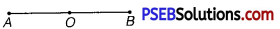A particle is in linear simple harmonic motion between the endpoints
(a) At the extreme point A, the particle is at rest momentarily. Hence, its velocity is zero at this point.
Its acceleration is positive as it is directed along with AO.
Force is also positive in this case as the particle is directed rightward.

(b) At the extreme point B, the particle is at rest momentarily. Hence, its velocity is zero at this point.
Its acceleration is negative as it is directed along B.
Force is also negative in this case as the particle is directed leftward.

(c)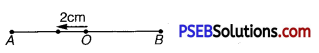The particle is extending a simple harmonic motion. O is the mean position of the particle. Its velocity at the mean position O is the maximum. The value for velocity is negative as the partide Is directed leftward. The acceleration and force of a particle executing SHM is zero at the mean position.

(d)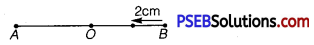The particle is moving towards point O from the end B. This direction of motion is opposite to the conventional positive direction, which is from A to R. Hence, the particle’s velocity and acceleration, and the force on it are all negative.

(e)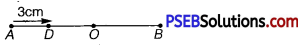The particle is moving towards point O from the end A. This direction of motion is from A to B, which is the conventional positive direction. Hence, the value for velocity, acceleration, and force are all positive.

(f)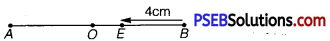This case is similar to the one given in (d).

Question 6.
Whi ch of the following relationships between the acceleration a and the displacement x of a particle involves simple harmonic motion?
(a) a=0.7x
(b) a=-200x2
(c) a= – 10 x (d) a=100x3
Solution:
A motion represents simple harmonic motion if it is governed by the force law:
F=-kx
ma’= -kx
∴ a = – $$\frac{k}{m}$$ x

where F is the force
m is the mass (a constant for a body)
x is the displacement
a is the acceleration.
k is a constant
Among the given equations, only equation a = -10 x is written in the
above form with $$\frac{k}{m}$$ =10. Hence, this relation represents SHM.Question 7.
The motion of a particle executing simple harmonic motion is described by the displacement function,
x(t) = A cos (ωt+Φ)
If the initial (t = 0) position of the particle is 1 cm and its initial velocity is ω cm/s, what are its amplitude and initial phase angle? The angular frequency of the particle is πs-1. If instead of the cosine function, we choose the sine function to describe the SHM:x = B sin(ωt + α), what are the amplitude and initial phase of the particle with the above initial conditions.
Solution:
Initially, at t = 0:
Displacement, x = 1 cm Initial velocity, ν = ω cm/s.
Angular frequency, ω = π rad s-1
It is given that:
x(t) = Acos (ωt+Φ)
1 = Acos(ω x 0 +Φ) = AcosΦ
AcosΦ =1 ……………………………….. (i)

Velocity, ν = $$\frac{d x}{d t}$$
ω = -Aω sin(ωt +Φ)
1 = -Asin(ω x 0 +Φ) = -AsinΦ
Asin Φ = -1 ………………………… (ii)
Squaring and adding equations (i) and (ii), we get
A2(sin2Φ +cos2Φ) = 1+1
A2 = 2
∴ A = $$\sqrt{2}$$ cm

Dividing equation (ii) by equation (i), we get
tanΦ = -1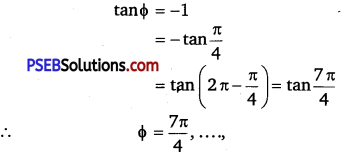SHM is given as
x = Bsin(ωt + α)
Putting the given values in this equation, we get 1 =B sin (ωt + α)
B sin α =1 …………………………… (iii)

Velocity, ν = $$\frac{d x}{d t}$$
ω =(ωB cos (ωt + a)
1 =B cos (ω x 0+α ) = B cos α …………………………………… (iv)
Squaring and adding equations (iii) and (iv), we get
B2 [sin2α +cos2 α] =1+1
B2 =2
B = $$\sqrt{2}$$ cm
Dividing equation (iii) by equation (iv), we get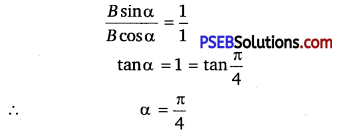Question 8.
A spring balance has a scale that reads from 0 to 50kg. The length of the scale is 20 cm. A body suspended from this balance, when displaced and released, oscillates with a period of 0.6 s. What is the weight of the body?
Solution:
Maximum mass that the scale can read, M = 50 kg
Maximum displacement of the spring = Length of the scale, l = 20 cm = 0.2 m Time period, T =0.6s
Maximum force exerted on the spring, F = Mg where,
g = acceleration due to gravity = 9.8 m/s2
F = 50 × 9.8 = 490 N
∴ Spring constant , K = $$\frac{F}{l}=\frac{490}{0.2}$$ = 2450Nm-1

Mass m, is suspended from the balance,
Time period, T = $$2 \pi \sqrt{\frac{m}{k}}$$
∴ m = $$\left(\frac{T}{2 \pi}\right)^{2} \times k=\left(\frac{0.6}{2 \times 3.14}\right)^{2} \times 2450$$ = 22.36 kg
∴ Weight of the body = mg = 22.36 x 9.8 = 219.167N
Hence, the weight of the body is about 219 N.

Question 9.
Aspringhavingwith aspiring constant 1200Nm-1 is mounted on a horizontal table as shown in figure. A mss of 3kg is attached to the free end of the spring. The mass is then pulled sideways to a distance of 2.0cm and released.Determine (i) the frequency of oscillations,
(ii) maximum acceleration of the mass, and
(iii) the maximum speed of the mass.
Solution:
Spring constant, k = 1200 Nm-1
mass,m = 3 Kg
Displacement,A = 2.0 cm = 0.02 m
(i) Frequency of oscillation y, is gyen by the relation
V = $$\frac{1}{T}=\frac{1}{2 \pi} \sqrt{\frac{k}{m}}$$
where, T is the time period
∴ v = $$\frac{1}{2 \times 3.14} \sqrt{\frac{1200}{3}}$$ = 3.18 s-1
Hence, the frequency of oscillations is 3.18 s-1.

(ii) Maximum acceleration a is given by the relation:
a = ω2A
where,
ω = Angular frequency = $$\sqrt{\frac{k}{m}}$$
A = Maximum displacement
∴ a = $$\frac{k}{m} A=\frac{1200 \times 0.02}{3}$$ = 8 ms-2
Hence, the maximum acceleration of the mass is 8.0 ms2

(iii) Maximum speed, νmax = Aω
= $$A \sqrt{\frac{k}{m}}=0.02 \times \sqrt{\frac{1200}{3}}$$ = 0.4 m/s
Hence, the maximum speed of the mass is 0.4 m/s.

Question 10.
In question 9, let us take the position of mass when the spring is unstretched as x =0, and the direction from left to right as the positive direction of x-axis. Give x as a function of time t for the oscillating massif at the moment we start the stopwatch (t = 0), the mass is
(a) at the mean position,
(b) at the maximum stretched position, and
(c) at the maximum compressed position.
In what way do these functions for SHM differ from each other, in frequency, in amplitude br the initial phase?
Solution:
(a) The functions have the same frequency and amplitude, but different initial phases.
Distance travelled by the mass sideways, A = 2.0 cm
Force constant of the spring, k =1200 N m-1
Mass, m =3kg
Angular frequency of oscillation,
ω = $$\sqrt{\frac{k}{m}}=\sqrt{\frac{1200}{3}}$$ = $$\sqrt{400}$$ = 20 rad s-1
When the mass is at the mean position, initial phase is 0.
Displacement,
x = A sinωt = 2 sin20 t

(b) At the maximum stretched position, the mass is toward the extreme right. Hence, the initial phase is $$\frac{\pi}{2}$$
Displacement, x = Asin $$\left(\omega t+\frac{\pi}{2}\right)$$
=2sin$$\left(20 t+\frac{\pi}{2}\right)$$
= 2 cos 20t

(c) At the maximum compressed position, the mass is toward the extreme left. Hence, the initial phase is $$\frac{3 \pi}{2}$$
Displacement, x = A sin $$\left(\omega t+\frac{3 \pi}{2}\right)$$
= 2sin $$\left(20 t+\frac{3 \pi}{2}\right)$$ = -2cos 20t
The functions have the same frequencyl $$\left(\frac{20}{2 \pi} \mathrm{Hz}\right)$$ land amplitude (2cm),
but different initial phases $$\left(0, \frac{\pi}{2}, \frac{3 \pi}{2}\right)$$Question 11.
Figures correspond to two circular motions. The radius of the circle, the period of revolution, the initial position, and the sense of revolution (i. e., clockwise or anti-clockwise) are indicated on each figure.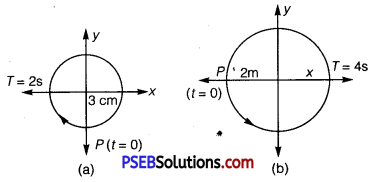Obtain the corresponding simple harmonic motions of the x-projection of the radius vector of the revolving particle P, in each case.
solution:
Time period,T =2s
Amplitude, A = 3cm
At time, t = O, the radius vector OP makes an angle $$\frac{\pi}{2}$$ with the positive x -axis, i.e., phase angle Φ = + $$\frac{\pi}{2}$$
Therefore, the equation of simple harmonic motion for the x —projection of OP, at time t, is given by the displacement equation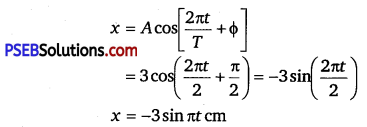(b) Time period, T = 4s
Amplitude, a =2 m
At time t = 0, OP makes an angle ir with the x-axis, in the anticlockwise direction. Hence, phase angle, Φ = +π
Therefore, the equation of simple harmonic motion for the x -projection of OP, at time t, is given as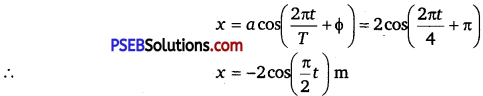Question 12.
Plot the corresponding reference circle for each of the following simple harmonic motions. Indicate the initial (t = 0) position of the particle, the radius of the circle, and the angular speed of the rotating particle. For simplicity, the sense of rotation may be fixed to be anticlockwise in every case: (x is in cm and t is in s).
(a) x= – 2sin(3t+$$\pi / \mathbf{3}$$ )
(b) x=cos ($$\pi / 6$$ – t)
(c) x=3 sin (2πt + $$\pi / 4$$ )
(d) x=2 cos πt
Solution:
(a) x = -2 sin $$\left(3 t+\frac{\pi}{3}\right)=+2 \cos \left(3 t+\frac{\pi}{3}+\frac{\pi}{2}\right)=2 \cos \left(3 t+\frac{5 \pi}{6}\right)$$

If this equation is compared with the standard SHM equation,
x =A cos $$\left(\frac{2 \pi}{T} t+\phi\right)$$ then we get
Amplitude, A = 2cm
Phase angle, Φ = $$\frac{5 \pi}{6}$$ =150°
Angular velocity, ω = $$\frac{2 \pi}{T}$$ =3 rad/sec
The motion of the particle can be pokted as shown in the following figure.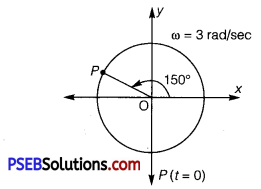(b) x=cos $$\left(\frac{\pi}{6}-t\right)$$ =cos $$\left(t-\frac{\pi}{6}\right)$$
If this equation is compared with the standard SHM equation,
x = A cos $$\left(\frac{2 \pi}{T} t+\phi\right)$$ then we get
Amplitude, A = 1 cm
Phase angle, Φ = $$-\frac{\pi}{6}$$ = – 30°
Angular velocity, ω = $$\frac{2 \pi}{T}$$ =1 rad/s
The motion of the particle can be plotted as shown in the following figure.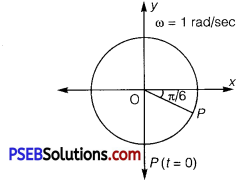(c) x =3sin $$\left(2 \pi t+\frac{\pi}{4}\right)$$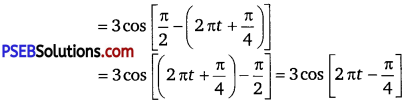If this equation is compared with the standard SHM equation
x = Acos $$\left(\frac{2 \pi}{T} t+\phi\right)$$ then we get
Amplitude, A = 3cm
Phase angle, Φ = $$-\frac{\pi}{4}$$
Angular velocity, ω = $$\frac{2 \pi}{T}$$ = 2π rad/s
The motion of the particle can be plotted as shown in the following figure.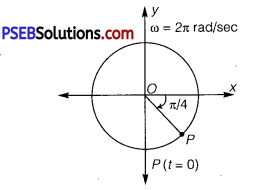(d) x=2cosπt
If this equation is compared with the standard SHM equation,
x = A cos $$\left(\frac{2 \pi}{T} t+\phi\right)$$ then we get
Amplitude, A = 2cm
Phase angle, Φ = 0
Angular velocity, ω = π rad/s
The motion of the particle can be plotted as shown in the following figure.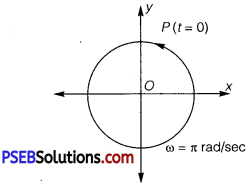Question 13.
Figure (a) shows a spring of force constant k clamped rigidly at one end and a mass m attached to its free end. A force F applied at the free end stretches the spring. Figure (b) shows the same spring with both ends free and attached to a mass m at either end. Each end of the spring in figure (b) is stretched by the same force F.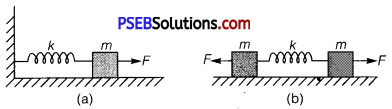(a) What is the minimum extension of the spring in the two cases?
(b) If the mass in Fig. (a) and the two masses in Fig. (b) are released, what is the period of oscillation in each case?
Solution:
(a) For the one block system:
When a force F, is applied to the free end of the spring, an extension l, is produced. For the maximum extension, it can be written as:
F=kl
where k is the spring constant
Hence, the maximum extension produced in the spring, l = $$\frac{F}{k}$$
For the two blocks system:
The displacement (x) produced in this case is:
x = $$\frac{l}{2}$$
Net force, F = +2kx =2k $$\frac{l}{2}$$
∴ l = $$\frac{F}{k}$$

(b) For the one blocks system:
For mass (m) of the block, force is written as
F = ma = m $$\frac{d^{2} x}{d t^{2}}$$
where, x is the displacement of the block in time t
∴ m $$\frac{d^{2} x}{d t^{2}}$$ = -kx
It is negative because the direction of elastic force is opposite to the direction of displacement.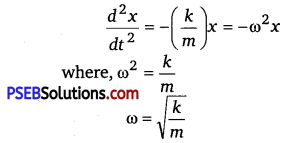where, ω is angular frequency of the oscillation
∴ Time period of the oscillation,
T= $$\frac{2 \pi}{\omega}=\frac{2 \pi}{\sqrt{\frac{k}{m}}}=2 \pi \sqrt{\frac{m}{k}}$$

For the two blocks system:
F=m $$\frac{d^{2} x}{d t^{2}}$$
m $$\frac{d^{2} x}{d t^{2}}$$ =-2kx

It is negative because the direction of elastic force is opposite to the direction of displacement.
$$\frac{d^{2} x}{d t^{2}}$$ = $$-\left[\frac{2 k}{m}\right] x$$ = – ω2x
where, Angular frequency, ω = $$\sqrt{\frac{2 k}{m}}$$
∴ Time period T = $$\frac{2 \pi}{\omega}=2 \pi \sqrt{\frac{m}{2 k}}$$Question 14.
The piston in the cylinder head of a locomotive has a stroke (twice the amplitude) of 1.0 in. If the piston moves with simple harmonic motion with an angular frequency of 200 rad/min, what is its maximum speed?
Solution:
Angular frequency of the piston, ω = 200 rad/min.
Stroke =1.0 m
Amplitude, A = $$\frac{1.0}{2}$$ = 0.5m
The maximum speed (νmax) of the piston is given by the relation
νmax =Aω = 200 x 0.5=100 m/min

Question 15.
The acceleration due to gravity on the surface of moon is 1.7 ms-2. What is the time period of a simple pendulum on the surface of moon If Its time period on the surface of earth is 3.5 s? (gon the surface of earth is 9.8 ms-2)
Solution:
Acceleration due to gravity on the surface of moon, g’ = 1.7m s-2
Acceleration due to gravity on the surface of earth, g = 9.8 ms-2
Time period of a simple pendulum on earth, T = 3.5 s
T= $$2 \pi \sqrt{\frac{l}{g}}$$

where l is the length of the pendulum
∴ l = $$\frac{T^{2}}{(2 \pi)^{2}} \times g=\frac{(3.5)^{2}}{4 \times(3.14)^{2}} \times 9.8 \mathrm{~m}$$

The length of the pendulum remains constant.
On Moon’s surface, time period,
T’ = $$2 \pi \sqrt{\frac{l}{g^{\prime}}}=2 \pi \sqrt{\frac{(3.5)^{2}}{\frac{4 \times(3.14)^{2}}{1.7}} \times 9.8}$$ = 8.4 s
Hence, the time period of the simple pendulum on the surface of Moon is 8.4 s.

Question 16.
(a) Time period of a particle in SHM depends on the force constant k and mass m of the particle:
T = $$2 \pi \sqrt{\frac{m}{k}}$$ A simple pendulum executes SHM approximately. Why then is the time period of a pendulum independent of the mass of the pendulum?

(b) The motion of a simple pendulum is approximately simple harmonic for small-angle oscillations. For larger angles of oscillation a more involved analysis shows that T is greater than $$2 \pi \sqrt{\frac{l}{g}}$$ Think of a qualitative argument to appreciate this result.

(c) A man with a wristwatch on his hand falls from the top of a tower. Does the watch give correct time during the free fall?

(d) What is the frequency of oscillation of a simple pendulum mounted in a cabin that is freely falling under gravity?
Solution :
(a) The time period of a simple pendulum, T = $$2 \pi \sqrt{\frac{m}{k}}$$
For a simple pendulum, k is expressed in terms of mass m, as
k ∝ m
$$\frac{m}{k}$$ = Constant
Hence, the time period T, of a simple pendulum is independent of the mass of the bob.

(b) In the case of a simple pendulum, the restoring force acting on the bob of the pendulum is given as
F = -mg sinθ
where, F = Restoring force; m = Mass of the bob; g = Acceleration due to
gravity; θ = Angle of displacement
For small θ, sinθ ≈ θ
For large 0,sin0 is greater than 0.
This decreases the effective value of g.
Hence, the time period increases as
T = $$2 \pi \sqrt{\frac{l}{g}}$$
where, l is the length of the simple pendulum

(c) The time shown by the wristwatch of a man falling from the top of a tower is not affected by the fall. Since a wristwatch does not work on the principle of a simple pendulum, it is not affected by the acceleration due to gravity during free fall. Its working depends on spring action.

(d) When a simple pendulum mounted in a cabin falls freely under gravity, its acceleration is zero. Hence the frequency of oscillation of this simple pendulum is zero.

Question 17.
A simple pendulum of length l and having a bob of mass M is suspended in a car. The car is moving on a circular track of radius R with a uniform speed v. If the pendulum makes small f oscillations in a radial direction about its equilibrium position, what will be its time period?
Solution:
The bob of the simple pendulum will experience the acceleration due to gravity and the centripetal acceleration provided by the circular motion of the car.
Acceleration due to gravity = g
Centripetal acceleration = $$\frac{v^{2}}{R}$$
where, v is the uniform speed of the car R is the radius of the track
Effective acceleration (aeff) is given as
aeff = $$\sqrt{g^{2}+\left(\frac{v^{2}}{R}\right)^{2}}$$

Time period, T = $$2 \pi \sqrt{\frac{l}{a_{e f f}}}$$
where, l is the length of the pendulum
∴ Time period, T = $$2 \pi \sqrt{\frac{l}{g^{2}+\frac{v^{4}}{R^{2}}}}$$

Question 18.
A cylindrical piece of cork of density of base area A and height h floats in a liquid of density ρl. The cork is depressed slightly and then released. Show that the cork oscillates up and down simple harmonically with a period
T = $$2 \pi \sqrt{\frac{\boldsymbol{h} \rho}{\rho_{\boldsymbol{l}} \boldsymbol{g}}}$$
where ρ is the density of cork. (Ignore damping due to viscosity of the liquid).
Solution:
Base area of the cork = A
Height of the cork = h
Density of the liquid = ρl
Density of the cork = ρ

In equilibrium:
Weight of the cork = Weight of the liquid displaced by the floating cork Let the cork be depressed slightly by x. As a result, some extra water of a certain volume is displaced. Hence, an extra up-thrust acts upward and provides the restoring force to the cork.
Up-thrust = Restoring force, F = Weight of the extra water displaced
F = -(Volume x Density x g)
Volume = Area x Distance through which the cork is depressed Volume = Ax
∴ F = -Ax ρlg ………………………… (i)
According to the force law,
F = kx
k = $$\frac{F}{x}$$

where k is a constant
k = $$\frac{F}{x}$$ = -Aρlg ………………………………. (ii)
The time period of the oscillations of the cork,
T = $$2 \pi \sqrt{\frac{m}{k}}$$ …………………………………… (iii)

where,
m = Mass of the cork
= Volume of the cork x Density
= Base area of the cork x Height of the cork x Density of the cork = Ahρ
Hence, the expression for the time period becomes
T = $$2 \pi \sqrt{\frac{A h \rho}{A \rho_{l} g}}$$ = 2$$\pi \sqrt{\frac{h \rho}{\rho_{l} g}}$$

Question 19.
One end of a U-tube containing mercury is connected to a suction pump and the other end to atmosphere. A small pressure difference is maintained between the two columns. Show that, when the suction pump is removed, the column of mercury in the U-tube executes simple harmonic motion.
Solution:
Area of cross-section of the U-tube = A
Density of the mercury column = ρ
Acceleration due to gravity = g
Restoring force, F = Weight of the mercury column of a certain height
F = -(Volume x Density x g)
F = -(A x 2h x ρ x g) = -2Aρgh = -k x Displacement in one of the arms (h)

where, 2h is the height of the mercury column in the two arms
k is a constant, given by k = $$-\frac{F}{h}$$ = 2Aρg
Time period = $$2 \pi \sqrt{\frac{m}{k}}=2 \pi \sqrt{\frac{m}{2 A \rho g}}$$
where, m is the mass of the mercury column
Let l be the length of the total mercury in the U-tube.
Mass of mercury, m = Volume of mercury x Density of mercury = Alρ
∴ T = $$2 \pi \sqrt{\frac{A l \rho}{2 A \rho g}}=2 \pi \sqrt{\frac{l}{2 g}}$$

Hence the mercury column executes simple harmonic motion with time period $$2 \pi \sqrt{\frac{l}{2 g}}$$

Question 20.
An air chamber of volume V has a neck area of cross-section a into which a ball of mass m just fits and can move up and down without any friction (see figure). Show that when the ball is pressed down a little and released, it executes SHM. Obtain an expression for the time period of oscillations assuming pressure-volume variations of air to be isothermal.Solution:
Volume of the air chamber = V
Area of cross-section of the neck = a
Mass of the ball = m
The pressure inside the chamber is equal to the atmospheric pressure. Let the ball be depressed by x units. As a result of this depression, there would be a decrease in the volume and an increase in the pressure inside the chamber.
Decrease in the volume of the air chamber, ΔV = ax
Volumetric strain =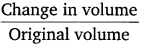⇒ $$\frac{\Delta V}{V}=\frac{a x}{V}$$

Bulk Modulus of air, B = $$\frac{\text { Stress }}{\text { Strain }}=\frac{-p}{\frac{a x}{V}}$$
In this case, stress is the increase in pressure. The negative sign indicates that pressure increases with a decrease in volume.
p = $$\frac{-B a x}{V}$$
The restoring force acting on the ball,
F = p × a = $$\frac{-B a x}{V} \cdot a=\frac{-B a^{2} x}{V}$$ ……………………………. (i)
In simple harmonic motion, the equation for restoring force is
F = -kx …………………………………….. (ii)
where, k is the spring constant Comparing equations (i) and (ii), we get
k = $$\frac{B a^{2}}{V}$$
Time period, T = $$2 \pi \sqrt{\frac{m}{k}}=2 \pi \sqrt{\frac{V m}{B a^{2}}}$$Question 21.
You are riding in an automobile of mass 3000kg. Assuming that you are examining the oscillation characteristics of its suspension system. The suspension sags 15 cm when the entire automobile is placed on it. Also, the amplitude of oscillation decreases by 50% during one complete oscillation. Estimate the values of (a) the spring constant k and (6) the damping constant b for the spring and shock absorber system of one wheel, assuming that each wheel supports 750 kg.
Solution:
Mass of the automobile, m = 3000 kg
Displacement in the suspension system, x = 15cm = 0.15 m
There are 4 springs in parallel to the support of the mass of the automobile.
The equation for the restoring force for the system:
F = -4 kx = mg

where, k is the spring constant of the suspension system
Time period, T = $$2 \pi \sqrt{\frac{m}{4 k}}$$
and, k = $$\frac{m g}{4 x}=\frac{3000 \times 10}{4 \times 0.15}$$ = 50000 = 5 x 10 4N/m
Spring constant, k = 5 x 104 N/m

Each wheel supports a mass, M = $$\frac{3000}{4}$$ = 750 kg
For damping factor b, the equation for displacement is written as:
x = x0e-bt/2M

The amplitude of oscillation decreases by 50%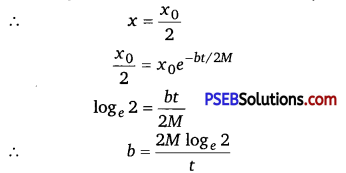where, Time petiod,t =$$2 \pi \sqrt{\frac{m}{4 k}}=2 \pi \sqrt{\frac{3000}{4 \times 5 \times 10^{4}}}$$ =0.7691s
∴ b =$$\frac{2 \times 750 \times 0.693}{0.7691}$$ =1351.58kg/s
Therefore, the damping constant of the spring is 1351.58 kg/s.

Question 22.
Show that for a particle in linear SHM the average kinetic energy over a period of oscillation equals the average potential energy over the same period.
Solution:
The equation of displacement of a particle executing SHM at an instant t is given as
x = Asinωt
where,
A = Amplitude of oscillation
ω = Angular frequency = $$\sqrt{\frac{k}{M}}$$
The velocity of the particle is
ν = $$\frac{d x}{d t}$$ = Aωcosωt

The kinetic energy of the particle is
Ek = $$\frac{1}{2} M v^{2}=\frac{1}{2} M A^{2} \omega^{2} \cos ^{2} \omega t$$
The potential energy of the particle is
Ep = $$\frac{1}{2} k x^{2}=\frac{1}{2} M \omega^{2} A^{2} \sin ^{2} \omega t$$

For time period T, the average kinetic energy over a single cycle is given as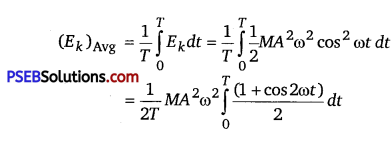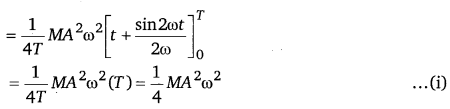And, average potential energy over one cycle is given as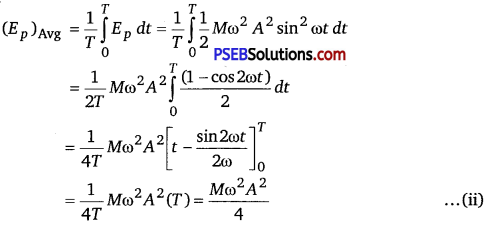It can be inferred from equations (i) and (ii) that the average kinetic energy for a given time period is equal to the average potential energy for the same time period.

Question 23.
A circular disc of mass 10 kg is suspended by a wire attached to its center. The wire is twisted by rotating the disc and released. The period of torsional oscillations is found to be 1.5s. The radius of the disc is 15 cm. Determine the torsional spring constant of the wire. (Torsional spring constant a is defined by the relation J = -αθ, where J is the restoring couple and 0 the angle of twist).
Solution:
Mass of the circular disc, m = 10 kg
Radius of the disc, r = 15cm = 0.15 m
The torsional oscillations of the disc has a time period, T = 1.5 s The moment of inertia of the disc is
I = $$\frac{1}{2}$$ mr2 = $$\frac{1}{2} \times(10) \times(0.15)^{2}$$ = 0.1125kg-m2

Time period, T = $$2 \pi \sqrt{\frac{I}{\alpha}}$$
where, α is the torsional constant.
An21 4 x(3.14)2x 0.1125 , M
α = $$\frac{4 \pi^{2} I}{T^{2}}=\frac{4 \times(3.14)^{2} \times 0.1125}{(1.5)^{2}}$$ = 1.972 N-m/rad
Hence, the torsional spring constant of the wire is 1.972 N-m rad-1.

Question 24.
A body describes simple harmonic motion with amplitude of 5 cm and a period of 0.2 s. Find the acceleration and velocity of the body when the displacement is (a) 5 cm, (b) 3 cm, (c) 0 cm.
Solution:
Amplitude, A = 5 cm = 0.05m
Time period, T = 0.2 s
(a) For displacement, x = 5 cm = 0.05m
Acceleration is given by
a = -ω2x = $$-\left(\frac{2 \pi}{T}\right)^{2} x=-\left(\frac{2 \pi}{0.2}\right)^{2} \times 0.05$$
Velocity is given by
ν = ω $$\sqrt{A^{2}-x^{2}}=\frac{2 \pi}{T} \sqrt{(0.05)^{2}-(0.05)^{2}}$$ = 0
When the displacement of the body is 5 cm, its acceleration is -5π2 m/s2 and velocity is 0.

(b) For displacement, x =3 cm = 0.03 m
Acceleration is given by
a = – ω2x = – $$\left(\frac{2 \pi}{T}\right)^{2}$$x = $$\left(\frac{2 \pi}{0.2}\right)^{2}$$ 0.03 = -3π2 m/s2
Velocity is given by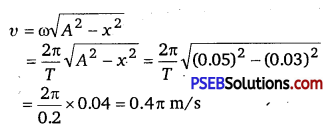When the displacement of the body is 3 cm, its acceleration is -3π m/s2 and velocity is 0.4π m/s.

(c) For displacement, x = 0
Acceleration is given by
a = – ω2x = 0
Velocity is given by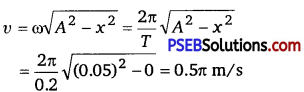When the displacement of the body is 0, its acceleration is 0, and velocity is0.5π m/s.Question 25.
A mass attached to a spring is free to oscillate, with angular velocity ω, in a horizontal plane without friction or damping. It is pulled to a distance x0 and pushed towards the center with a velocity v0 at time t = 0. Determine the amplitude of the resulting oscillations in terms of the parameters ω, x0, and v0. [Hint: Start with the equation x = a cos(ωt + θ) and note that the initial velocity is negative.]
Solution:
The displacement equation for an oscillating mass is given by
x = Acos(ωt + θ) …………………………… (i)
where A is the amplitude
x is the displacement
θ is the phase constant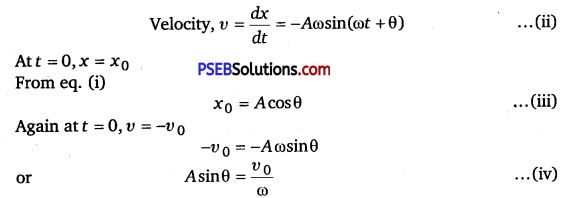Squaring and adding equations (iii) and (iv), we get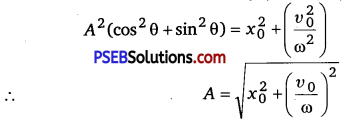Hence, the amplitude of the resulting oscillation is $$\sqrt{x_{0}^{2}+\left(\frac{v_{0}}{\omega}\right)^{2}}$$